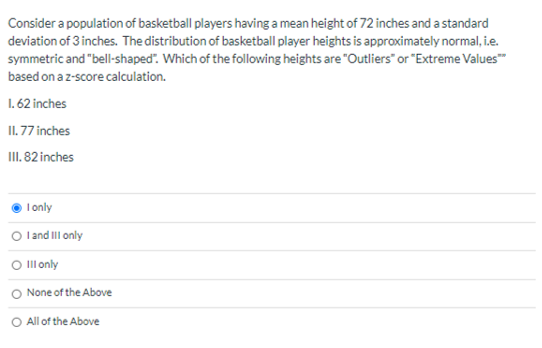# Consider a population of basketball players having a mean height of 72 inches and a standard deviation of 3 inches. The distribution of basketball player heights is approximately normal, i.e. symmetric and "bell-shaped". Which of the following heights are "Outliers" or "Extreme Values" based on a z-score calculation. 1. 62 inches II. 77 inches III. 82 inches I only O l and Ill only O Ill only None of the Above

Question

Consider a population of basketball players having a mean height of 72 inches and a standard deviation of 3 inches.  The distribution of basketball player heights is approximately normal, i.e. symmetric and “bell-shaped”.  Which of the following heights are “Outliers” or “Extreme Values”” based on a z-score calculation.

I. 62 inches

II. 77 inches

III. 82 incheshelp_outlineImage TranscriptioncloseConsider a population of basketball players having a mean height of 72 inches and a standard deviation of 3 inches. The distribution of basketball player heights is approximately normal, i.e. symmetric and "bell-shaped". Which of the following heights are "Outliers" or "Extreme Values" based on a z-score calculation. 1. 62 inches II. 77 inches III. 82 inches I only O l and Ill only O Ill only None of the Above fullscreen

### Want to see this answer and more?

Experts are waiting 24/7 to provide step-by-step solutions in as fast as 30 minutes!*

*Response times may vary by subject and question complexity. Median response time is 34 minutes for paid subscribers and may be longer for promotional offers.
Tagged in
Math
Statistics

### Measures